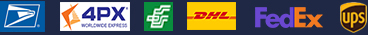•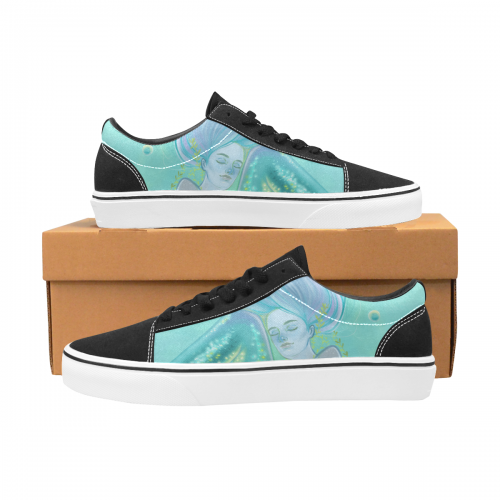•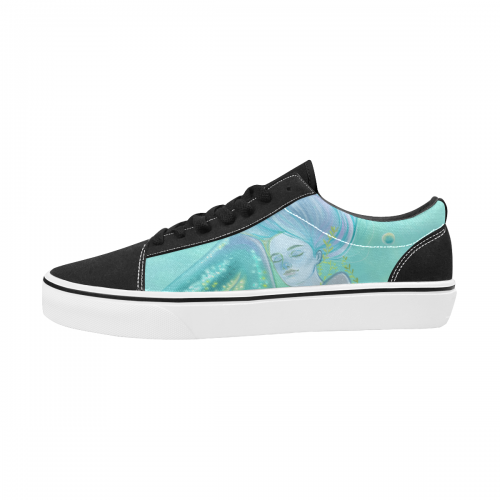•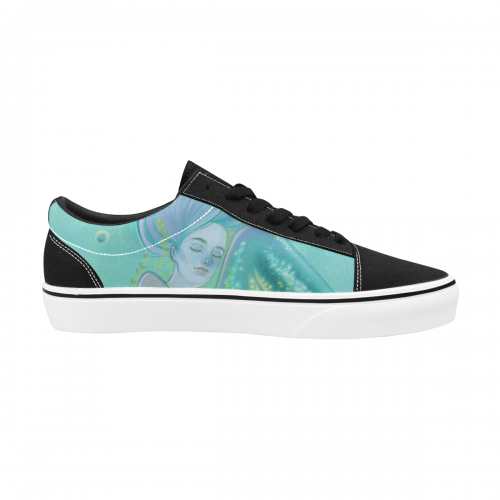•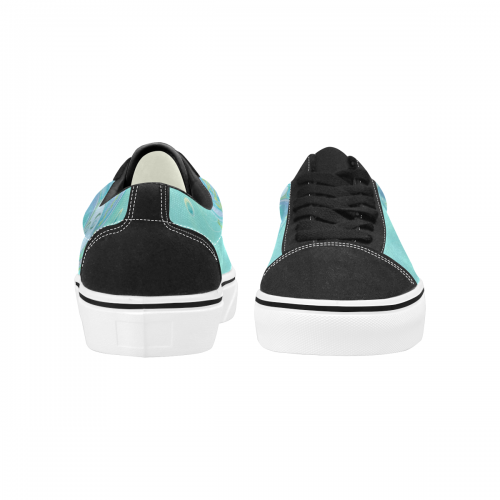### Women's Lace-Up Canvas Shoes (Model E001-2)

\$37.99 ~ \$38.99
Retail Price \$69.99 ~ \$69.99

Partial Shipping Included

•   Black

EUR35 = US4.5=UK3 =226mm =8.90inch
EUR36 = US5 =UK3.5 = 233mm = 9.17inch
EUR47 = US6 =UK4.5= 239mm =9.41inch

EUR38 = US6.5 =UK5 =246mm =9.70inch

EUR39 = US7.5 =UK6 = 253mm = 9.96inch

EUR40 = US8.5 =UK7= 259mm = 10.20inch
EUR41 = US9 =UK7.5 = 266mm =10.47inch
EUR42 = US9.5 =UK8 =273mm =10.74inch
EUR43 = US10 =UK8.5 =279mm = 10.98inch

Payment methods we accept: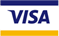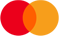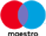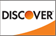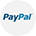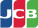We deliver with: